# A linear transformer is connected as shown below. Assume Vs1 = 10/0° V, ZL1 = j502,...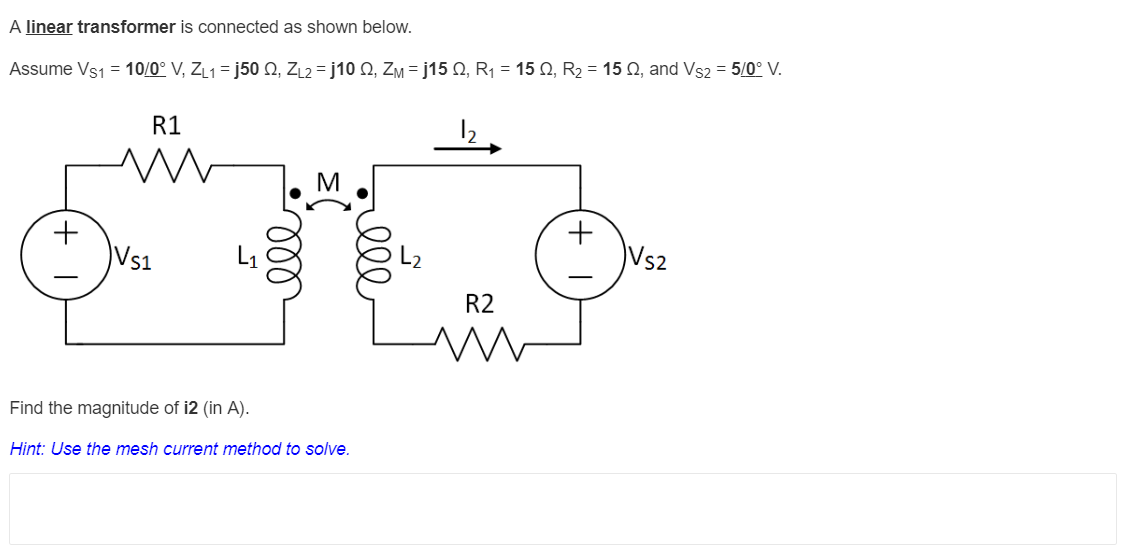A linear transformer is connected as shown below. Assume Vs1 = 10/0° V, ZL1 = j502, ZL2 = 310 2, ZM = 315 2, R1 = 152, R2 = 152, and Vs2 = 5/0° V. R1 M M + Vs1 ell Vs2 R2 Find the magnitude of i2 (in A). Hint: Use the mesh current method to solve.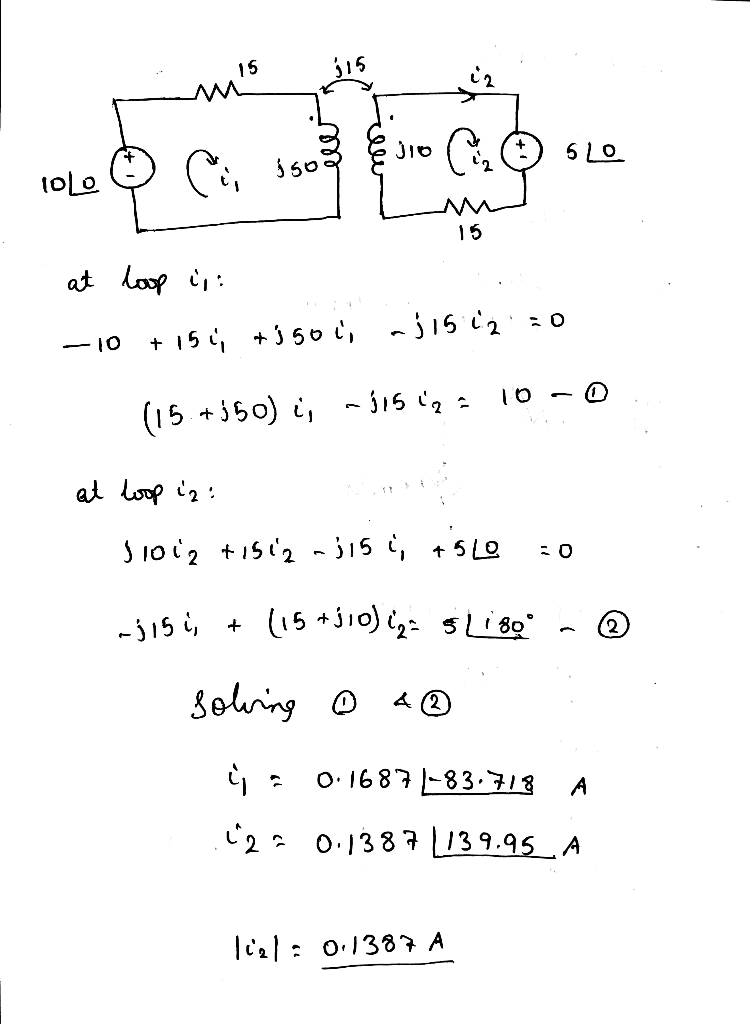##### Add Answer of: A linear transformer is connected as shown below. Assume Vs1 = 10/0° V, ZL1 = j502,...
Similar Homework Help Questions
• ### R2 R, Q2. In the above circuit, Vs1 = 11 V, Vs2 = 22 V, R1...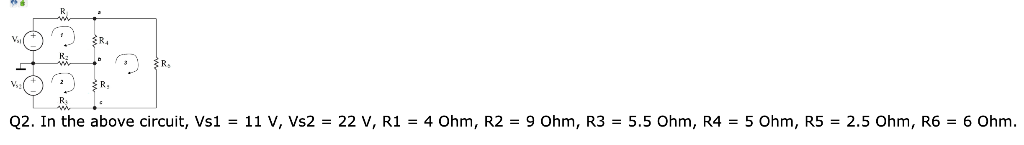R2 R, Q2. In the above circuit, Vs1 = 11 V, Vs2 = 22 V, R1 = 4 Ohm, R2 = 9 Ohm, R3 = 5.5 Ohm, R4 = 5 Ohm, R5 = 2.5 Ohm, R6 = 6 Ohm. B. Using mesh analysis, answer the following questions (Q2.2) Find the current through the resistor R1 in the direction from left to right. (Unit: A) Submit Answer Tries 0/3 (Q2.3) Find the current through the resistor R2 in the direction from...

• ### For the questions on this page, an ideal transformer is connected as shown below. Assume Vs...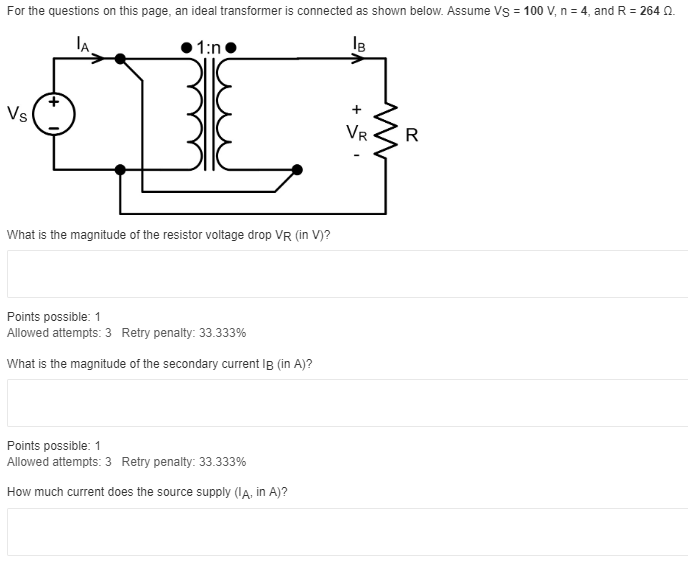For the questions on this page, an ideal transformer is connected as shown below. Assume Vs = 100 V, n = 4, and R = 264 0. 1:n IB Vs + VR R What is the magnitude of the resistor voltage drop VR (in V)? Points possible: 1 Allowed attempts: 3 Retry penalty: 33.333% What is the magnitude of the secondary current IB (in A)? Points possible: 1 Allowed attempts: 3 Retry penalty: 33.333% How much current does the source...

• ### An ideal transformer is connected as shown below. If Vs = 83 V, N1 = 7,...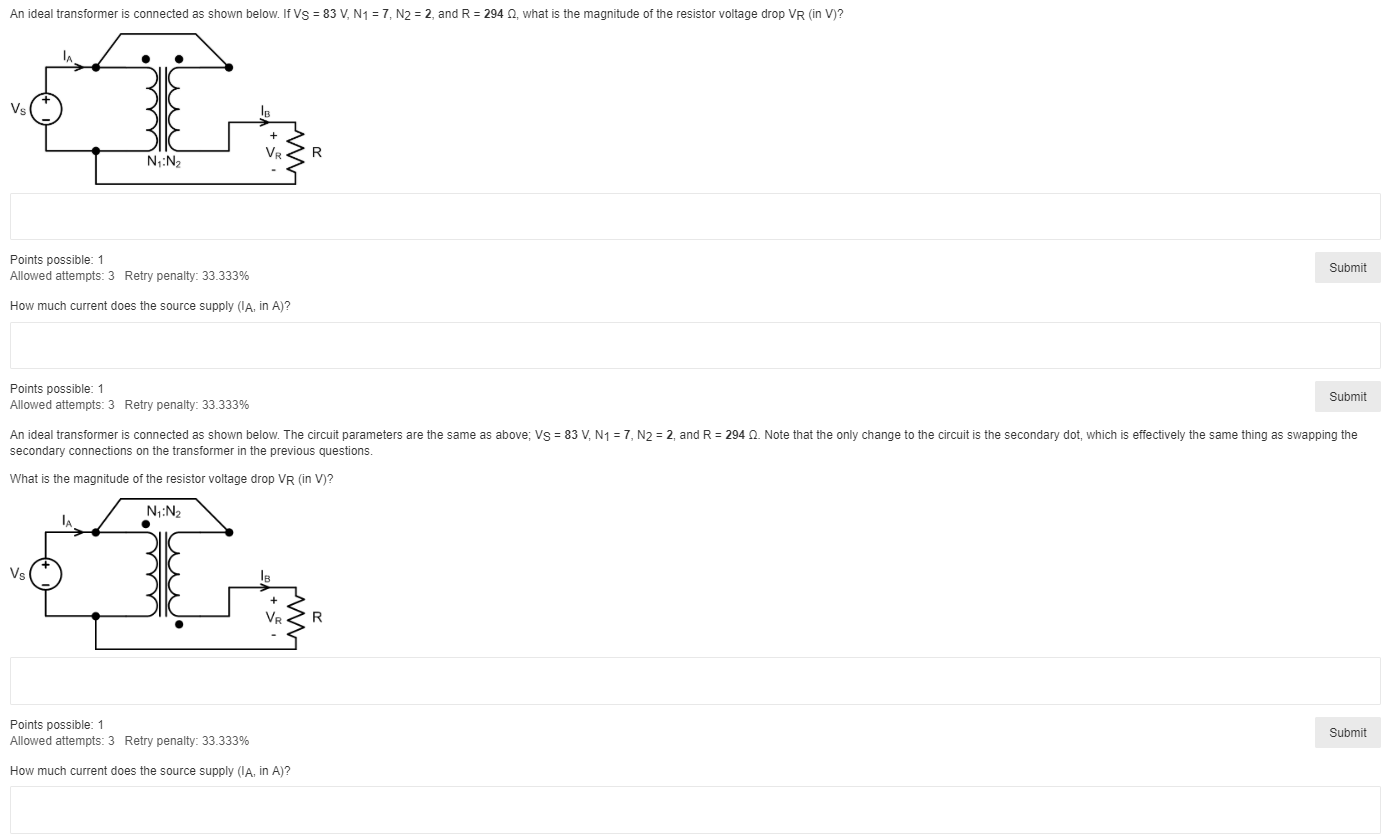An ideal transformer is connected as shown below. If Vs = 83 V, N1 = 7, N2 = 2, and R = 294 , what is the magnitude of the resistor voltage drop VR (in V)? Vs N:N Points possible: 1 Allowed attempts: 3 Retry penalty: 33.333% Submit How much current does the source supply (IA, in A)? Points possible: 1 Allowed attempts: 3 Retry penalty: 33.333% Submit An ideal transformer is connected as shown below. The circuit parameters are...

• ### An ideal transformer is connected as shown below. If Vs = 76 VRMS, R1 = 5370,...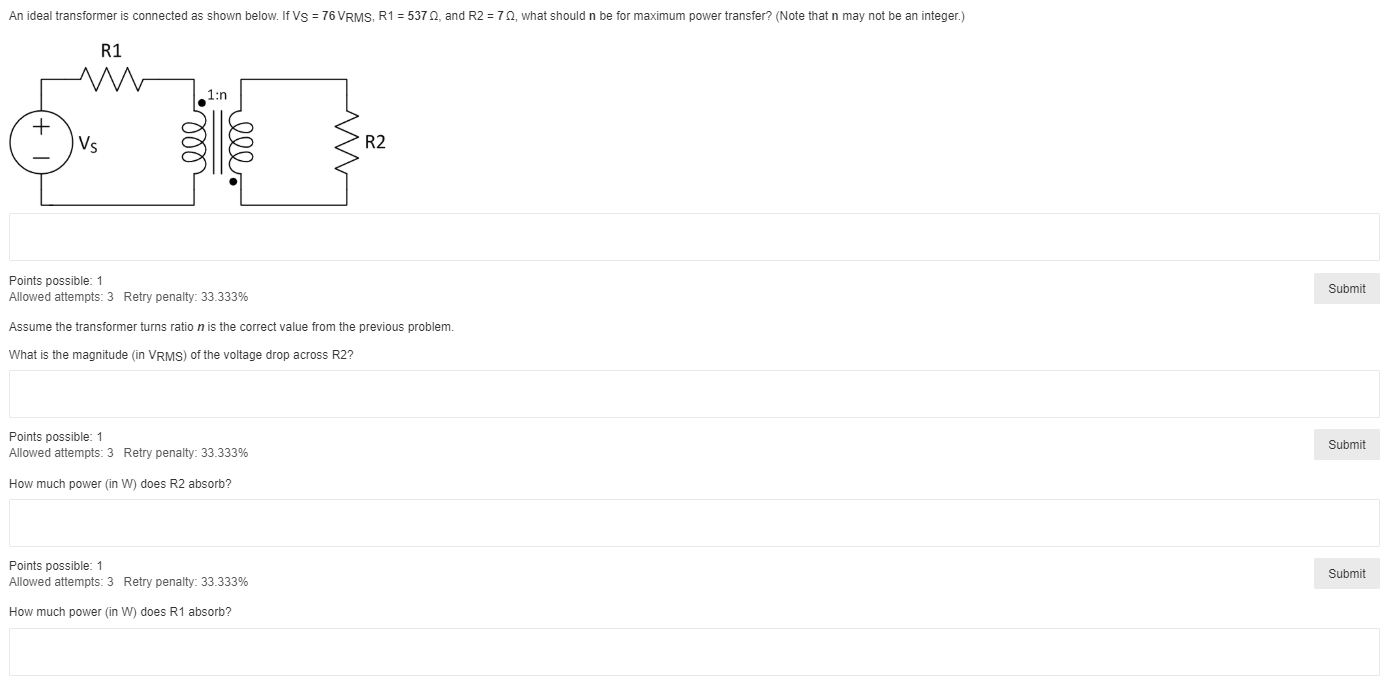An ideal transformer is connected as shown below. If Vs = 76 VRMS, R1 = 5370, and R2 = 72, what should n be for maximum power transfer? (Note that n may not be an integer.) R1 M 1:n + Vs llel R2 Points possible: 1 Allowed attempts: 3 Retry penalty: 33.333% Submit Assume the transformer turns ratio n is the correct value from the previous problem. What is the magnitude (in VRMS) of the voltage drop across R2? Points...

• ### Consider the following figure. (Assume V = 11 V, R1 = 1.9 Ω, and R2 =...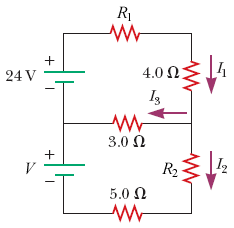Consider the following figure. (Assume V = 11 V, R1 = 1.9 Ω, and R2 = 1.2 Ω.) (a) Can the circuit shown above be reduced to a single resistor connected to the batteries? Explain. (b) Calculate each of the unknown currents I1, I2, and I3 for the circuit. (Give the magnitude of the current only.) I1 = A I2 = A I3 = A

• ### im not sure what to do wjrh the rest R R3 w RS ww Vsi R₂²...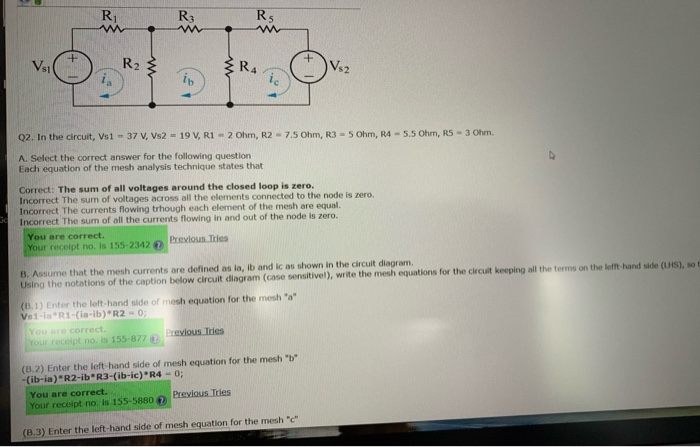im not sure what to do wjrh the rest R R3 w RS ww Vsi R₂² RA Vsa ia ib ic Q2. In the circuit, Vs1 - 37 V, Vs2 - 19 V, R1 - 2 Ohm, R2 - 7.5 Ohm, R35 Ohm, R4 - 5.5 Ohm, R5 - 3 Ohm. A. Select the correct answer for the following question Each equation of the mesh analysis technique states that Correct: The sum of all voltages around the closed loop is...

• ### 7. 1/4 points | Previous Answers SerCP11 18.4.P.027 My Notes Consider the following figure. (Assume V-15 V, R1-2.6 Ω, and R2-1.62.) RI 3.0 2 R2 5.0 Ω (a) Can the circuit shown above be reduced to a s...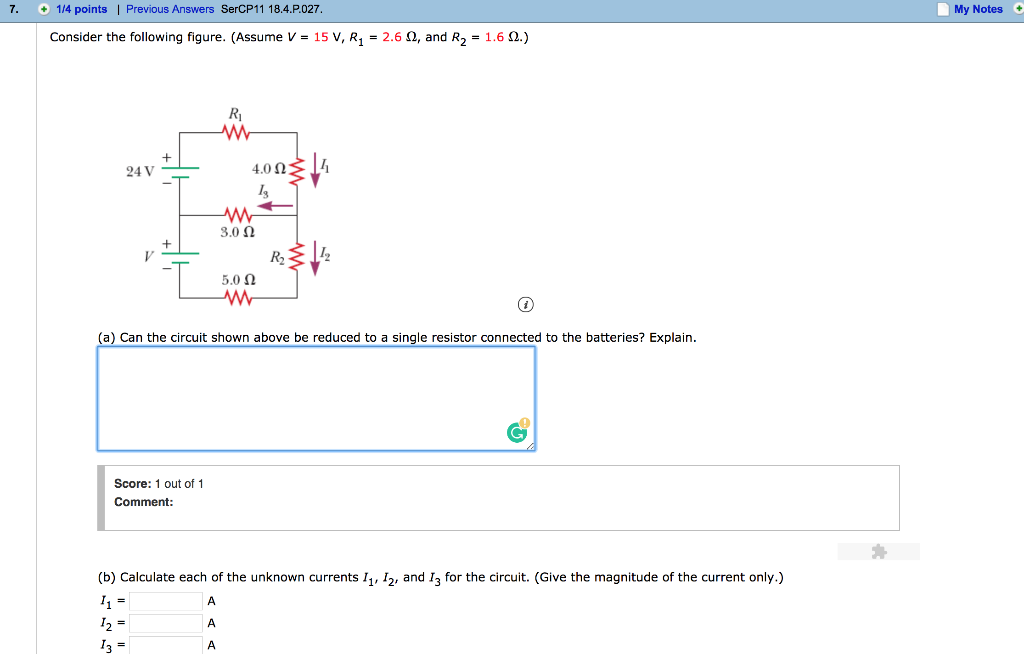7. 1/4 points | Previous Answers SerCP11 18.4.P.027 My Notes Consider the following figure. (Assume V-15 V, R1-2.6 Ω, and R2-1.62.) RI 3.0 2 R2 5.0 Ω (a) Can the circuit shown above be reduced to a single resistor connected to the batteries? Explain. Score: 1 out of 1 Comment: (b) Calculate each of the unknown currents 11, I2, and I3 for the circuit. (Give the magnitude of the current only.) 12 = 13- 7. 1/4 points | Previous Answers...

• ### From the circuit shown below, (a) use KCL and KVL to solve for the three currents....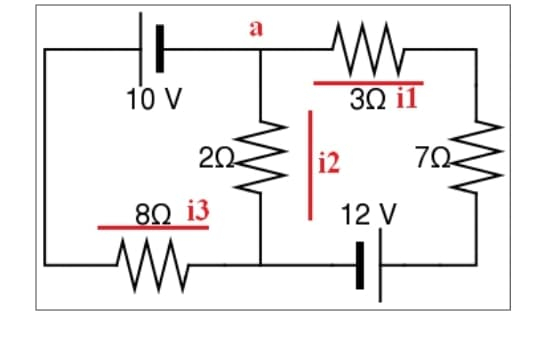From the circuit shown below, (a) use KCL and KVL to solve for the three currents. (b) With the given data below and the two currents flowing clockwise, determine both currents using mesh analysis and the voltages across each resistor. r1= 3 Ohms r2= 7 Ohms r3= 2 Ohms r4= 8 Ohms a M 10 V 30 il 222 i2 722 892 i3 12 V W

• ### Shown in the circuit below is a V= 10.0 V battery connected with three resistors and...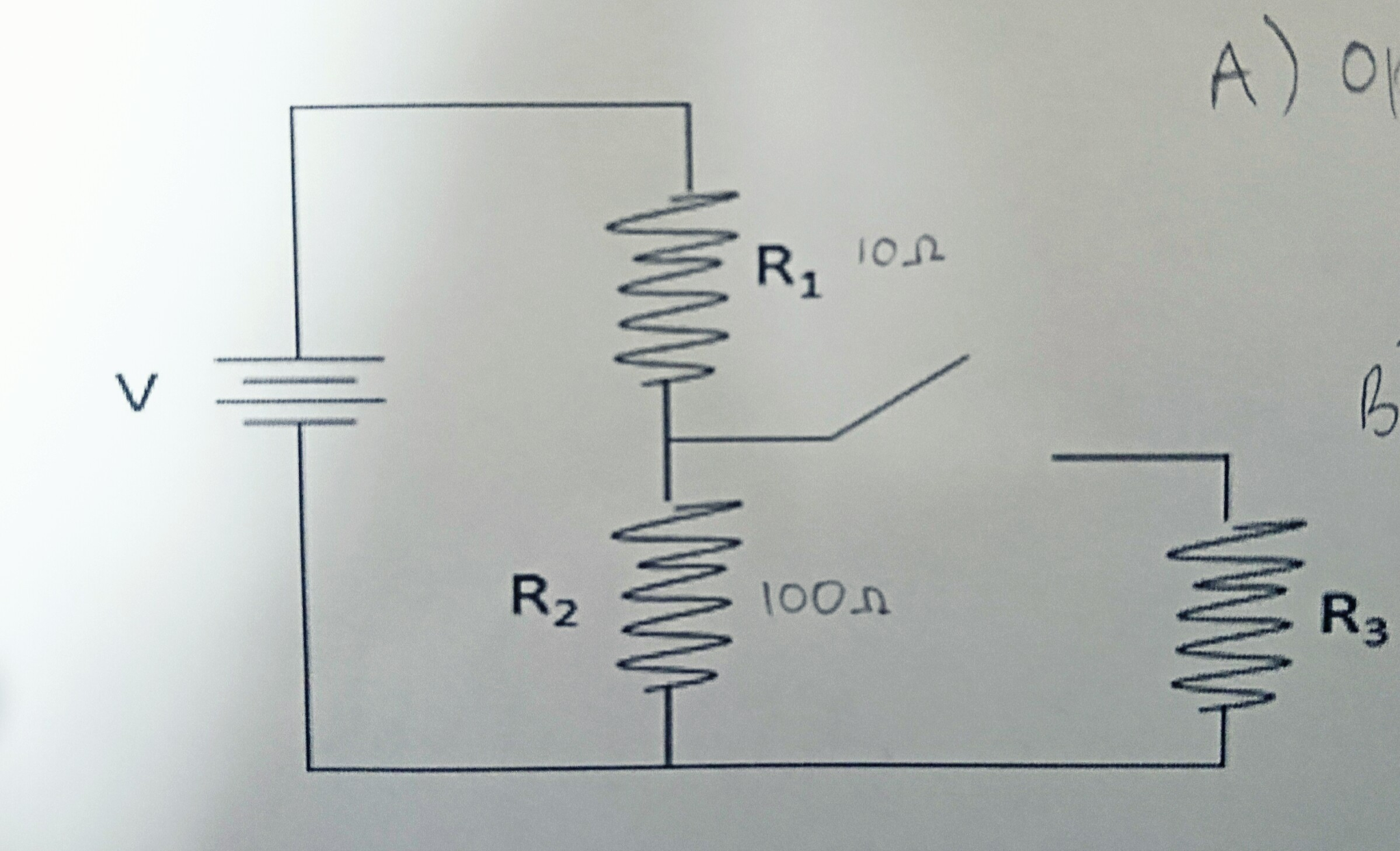Shown in the circuit below is a V= 10.0 V battery connected with three resistors and a switch. The resistances for each resistor are R1= 10 ohms, R2= 100 ohms, and R3= 1.0 ohms. B) Calculate the current through each resistor when the switch is closed. A) Calculate the equivalent resistance when 1) the switch is open and 2) when the switch is closed. E R, Ion 2 loon R

• ### Four resistors are connected in a circuit as shown below with a 12 V battery. a)...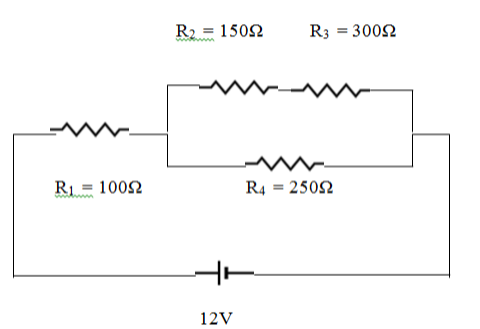Four resistors are connected in a circuit as shown below with a 12 V battery. a) What is the equivalent resistance of R2 and R3? b) What is the equivalent resistance of R2, R3, and R4? c) What is the equivalent resistance of the entire circuit? d) What is the total current flowing through the circuit? e) What is the voltage and current for each resistor? Resistor Voltage Current 100 Ohm 150 Ohm 300 Ohm 250 Ohm R2 = 150Ω...

Free Homework App